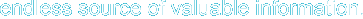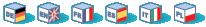# Introduction

PHP supports the direct io functions as described in the Posix Standard (Section 6) for performing I/O functions at a lower level than the C-Language stream I/O functions (fopen(), fread(),..). The use of the DIO functions should be considered only when direct control of a device is needed. In all other cases, the standard filesystem functions are more than adequate.

Note: This extension has been moved to the PECL repository and is no longer bundled with PHP as of PHP 5.1.0.

This extension is only available on Windows Platforms as of PHP 5.0.0

# Requirements

No external libraries are needed to build this extension.

# Installation

To get these functions to work, you have to configure PHP with --enable-dio.

# Predefined Constants

The constants below are defined by this extension, and will only be available when the extension has either been compiled into PHP or dynamically loaded at runtime.

c (integer)

F_DUPFD (integer)

F_GETFD (integer)

F_GETFL (integer)

F_GETLK (integer)

F_GETOWN (integer)

F_RDLCK (integer)

F_SETFL (integer)

F_SETLK (integer)

F_SETLKW (integer)

F_SETOWN (integer)

F_UNLCK (integer)

F_WRLCK (integer)

O_APPEND (integer)

O_ASYNC (integer)

O_CREAT (integer)

O_EXCL (integer)

O_NDELAY (integer)

O_NOCTTY (integer)

O_NONBLOCK (integer)

O_RDONLY (integer)

O_RDWR (integer)

O_SYNC (integer)

O_TRUNC (integer)

O_WRONLY (integer)

S_IRGRP (integer)

S_IROTH (integer)

S_IRUSR (integer)

S_IRWXG (integer)

S_IRWXO (integer)

S_IRWXU (integer)

S_IWGRP (integer)

S_IWOTH (integer)

S_IWUSR (integer)

S_IXGRP (integer)

S_IXOTH (integer)

S_IXUSR (integer)

# Runtime Configuration

This extension has no configuration directives defined in php.ini.

# Resource Types

One resource type is defined by this extension: a file descriptor returned by dio_open().